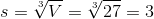## Example Questions

### Example Question #1 : How To Find The Length Of An Edge Of A Cube

Our backyard pool holds 10,000 gallons.  Its average depth is 4 feet deep and it is 10 feet long.  If there are 7.48 gallons in a cubic foot, how wide is the pool?

33  ft

7.48 ft

100 ft

30 ft

133 ft

33  ft

Explanation:

There are 7.48 gallons in cubic foot. Set up a ratio:

1 ft3 / 7.48 gallons = x cubic feet / 10,000 gallons

Pool Volume = 10,000 gallons = 10,000 gallons * (1 ft3/ 7.48 gallons) = 1336.9 ft3

Pool Volume = 4ft x 10 ft x WIDTH = 1336.9 cubic feet

Solve for WIDTH:

4 ft x 10 ft x WIDTH = 1336.9 cubic feet

WIDTH = 1336.9 / (4 x 10) = 33.4 ft

### Example Question #2 : How To Find The Length Of An Edge Of A Cube

A cube has a volume of 64cm3. What is the area of one side of the cube?

16cm3

16cm2

4cm2

4cm

16cm

16cm2

Explanation:

The cube has a volume of 64cm3, making the length of one edge 4cm (4 * 4 * 4 = 64).

So the area of one side is 4 * 4 = 16cm2

### Example Question #1 : How To Find The Length Of An Edge Of A Cube

A cube as the volume of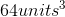.

Find the length of a side of this cube.Explanation:

The formula to find the volume of the cube is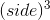Since we know the volume, we can set up the equation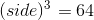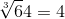### Example Question #2 : How To Find The Length Of An Edge Of A Cube

A cube has a surface area of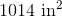, what is the length of the side of the cube? (If necessary, round to the nearest hundredth.)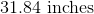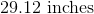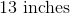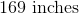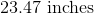Explanation:

To find the length of the side of a square given the surface area, use the surface area formula and solve for: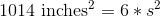, now divide both sides by 6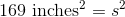, now square root both sides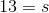.

### Example Question #3 : How To Find The Length Of An Edge Of A Cube

A certain cubic box when unfolded and laid flat on a table covers exactly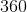square units of space. What is the width of the box, in units?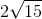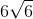Explanation:

To find the length of the edge of a cube from its surface area, remember that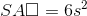, whereis the length of a side.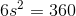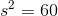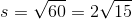So, the box isunits long.

### Example Question #4 : How To Find The Length Of An Edge Of A Cube

Given the volume of a cube is, find the side length.Explanation:

To find side length, simply realize that volume is the side length cubed. Thus,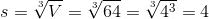### Example Question #5 : How To Find The Length Of An Edge Of A Cube

Find the length of the edge of a cube given the volume is.